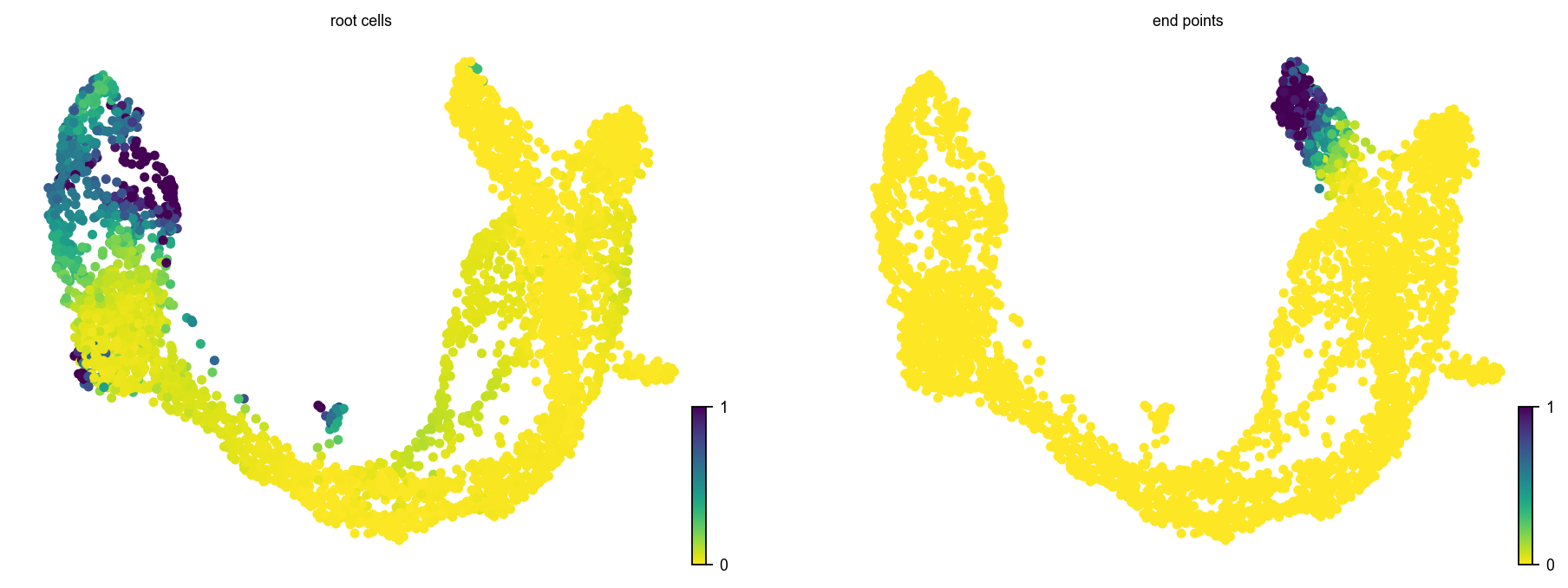# scvelo.tl.terminal_states¶

scvelo.tl.terminal_states(data, vkey='velocity', groupby=None, groups=None, self_transitions=False, eps=0.001, random_state=0, copy=False, **kwargs)

Computes terminal states (root and end points).

The end points and root cells are obtained as stationary states of the velocity-inferred transition matrix and its transposed, respectively, which is given by left eigenvectors corresponding to an eigenvalue of 1, i.e.

$μ^{\textrm{end}}=μ^{\textrm{end}} \pi, \quad μ^{\textrm{root}}=μ^{\textrm{root}} \pi^{\small \textrm{T}}.$
scv.tl.terminal_states(adata)Alternatively, we recommend to use cellrank.tl.terminal_states() providing an improved/generalized approach of identifying terminal states.
Parameters: data : AnnData Annotated data matrix. vkey : str (default: ‘velocity’) Name of velocity estimates to be used. groupby : str, list or np.ndarray (default: None) Key of observations grouping to consider. Only to be set, if each group is assumed to have a distinct lineage with an independent root and end point. groups : str, list or np.ndarray (default: None) Groups selected to find terminal states on. Must be an element of .obs[groupby]. To be specified only for very distinct/disconnected clusters. self_transitions : bool (default: False) Allow transitions from one node to itself. eps : float (default: 1e-3) Tolerance for eigenvalue selection. random_state : int or None (default: 0) Seed used by the random number generator. If None, use the RandomState instance by np.random. copy : bool (default: False) Return a copy instead of writing to data. **kwargs Passed to scvelo.tl.transition_matrix(), e.g. basis, weight_diffusion. Returns or updates data with the attributes root_cells (.obs) – sparse matrix with transition probabilities. end_points (.obs) – sparse matrix with transition probabilities.